# How to Use the Pythagoras Theorem: Tips and Tricks from a Maths Tutor

The Pythagoras Theorem is a maths rule that helps you find the length of the sides of a right-angled triangle. You need to know how to use the Pythagoras Theorem when you have a right-angled triangle (a triangle with one angle that is exactly 90 degrees) with the measurements of two sides and need to discover the length of one of the slides.

## What is the Pythagoras Theorem?

The Pythagoras Theorem states that the sum of the squares of the lengths of the two shorter sides of a right-angled triangle is equal to the square of the length of the longest side, known as the hypotenuse.

The theorem can be written mathematically as:

a² + b² = c²

Below, you can see a visual representation of the theorem: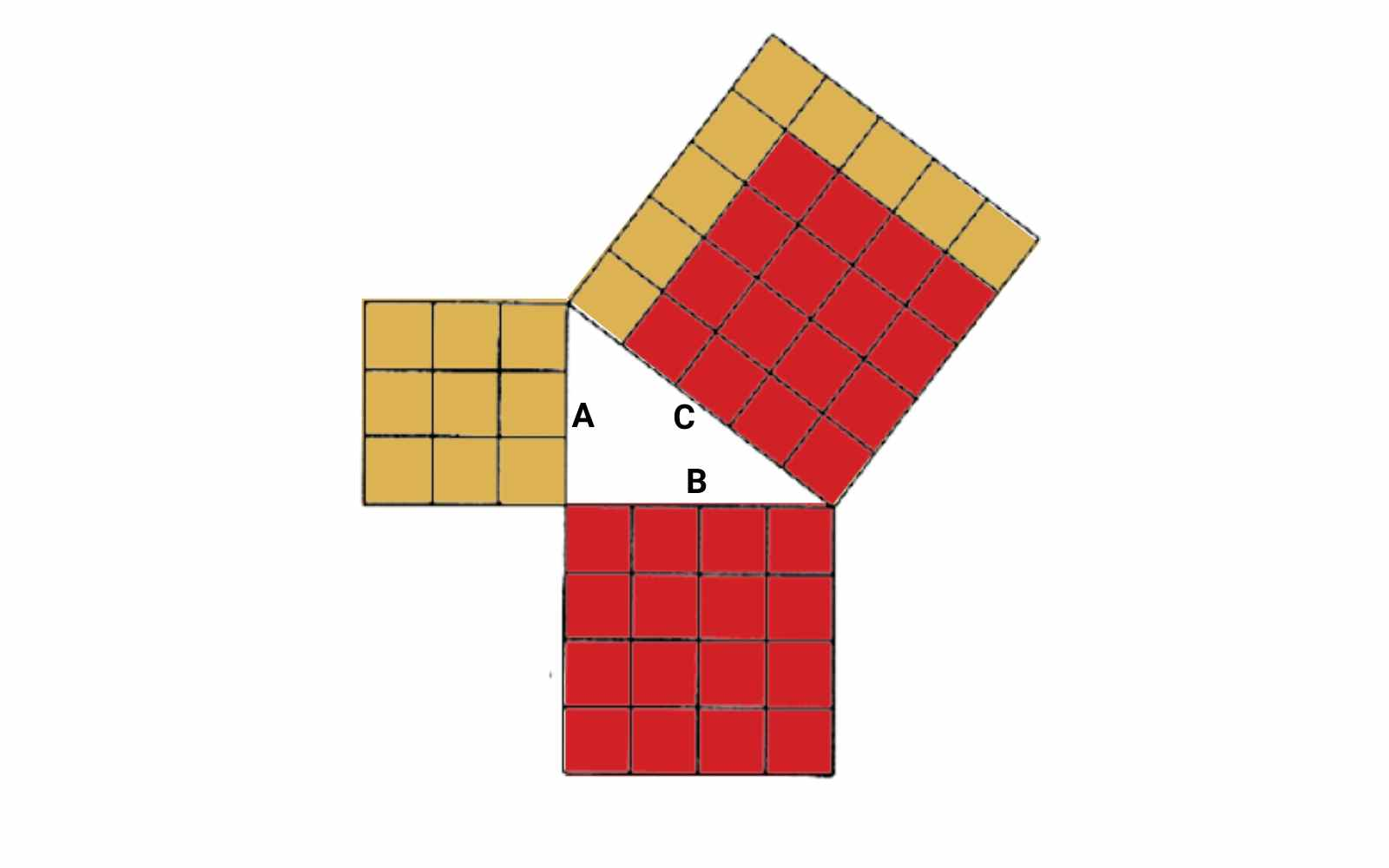This article will show you how to use the Pythagoras Theorem, but if you are still struggling with the concept, maths tutoring may be beneficial!

## When to Use the Pythagoras Theorem

The Pythagoras Theorem is an essential mathematical concept for right triangles that has a wide range of applications in many fields.

Here are some of the uses of the Pythagoras Theorem:

• Finding the length of the sides of a right-angled triangle: The Pythagoras Theorem helps you find the length of the hypotenuse (the longest side) or one of the shorter sides of a right-angled triangle, given the lengths of the other two sides.
• Checking whether a triangle is right-angled: If you know the lengths of the three sides of a triangle, you can use the Pythagoras Theorem to determine whether it is a right-angled triangle or not.
• Distance and displacement: The Pythagoras Theorem can be used to calculate the distance between two points in a two-dimensional space or the displacement between two points in a three-dimensional space.
• Navigation: The Pythagoras Theorem is used in navigation to calculate the distance between two points on a map or the distance between two cities on a globe.

The Pythagoras Theorem is a foundational concept that you need to understand to succeed in maths subjects. Mastering the Pythagoras Theorem will help you build a strong foundation in mathematics that you can build on for future maths classes.

## How to Use the Pythagoras Theorem

How to use the Pythagoras Theorem to find a missing side of a right triangle: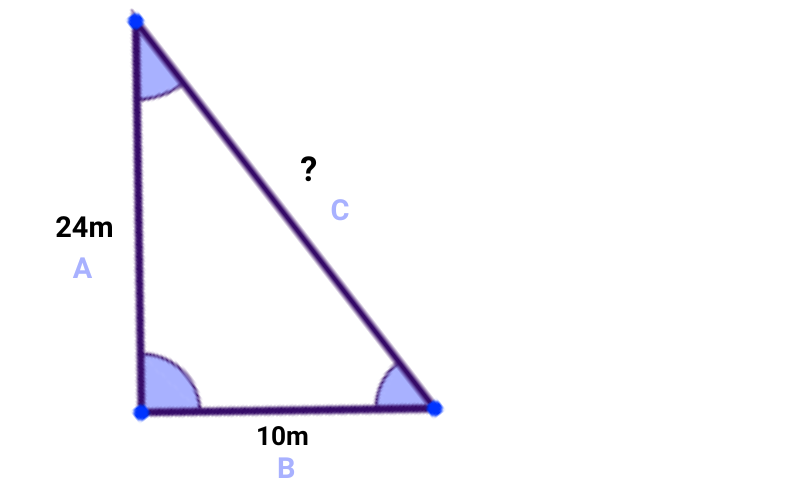a² + b² = c²

2. Put in what you know:

10² + 24² = c²

3. Calculate:

100 + 576 = c²

686 = c²

4. Swap sides:

c² = 686

5. Square root:

c = √686

c= 26.2

Therefore, the length of the hypotenuse is 26.2 cm.

## Pythagoras Theorem Examples

It is easiest to learn maths by working through examples!

Question 1: Find the length of the hypotenuse of a right-angled triangle with legs of length 3 cm and 4 cm.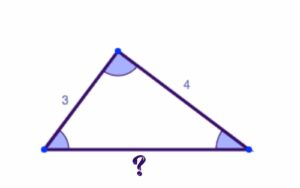Solution: Using the Pythagorean Theorem, we can find the length of the hypotenuse as follows:

• a² + b² = c²
• 3² + 4² = c²
• 9 + 16 = c²
• 25 = c²
• c = √25
• c = 5cm

Therefore, the length of the hypotenuse is 5 cm.

Question 2: Find the length of the shorter leg of a right-angled triangle with a hypotenuse of 10 cm and the longer leg of 8 cm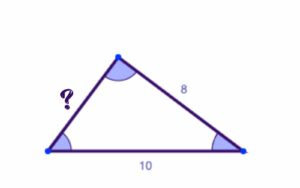Solution: Using the Pythagorean Theorem, we can find the length of the shorter leg as follows:

• a² + b² = c²
• a² + 8² = 10²
• a² + 64 = 100
• a² + 64 (-64) = 100 (-64)
• a² = 36
• a = √36 = 6 cm

Therefore, the length of the shorter leg is 6 cm.

Question 3: Determine whether the triangle with sides of length 5 cm, 12 cm, and 13 cm is a right-angled triangle.

Solution: We can use the Pythagorean Theorem to check whether the triangle is a right-angled triangle or not as follows:

• a² + b² = c²
• 5² + 12² = 13²
• 25 + 144 = 169
• 169 = 169

Since the equation is true, we can conclude that the triangle with sides of length 5 cm, 12 cm, and 13 cm is a right-angled triangle.

Question 4: A 15m ladder is placed against a wall so that the base of the ladder is 9m from the wall. What is the height at which the ladder touches the wall?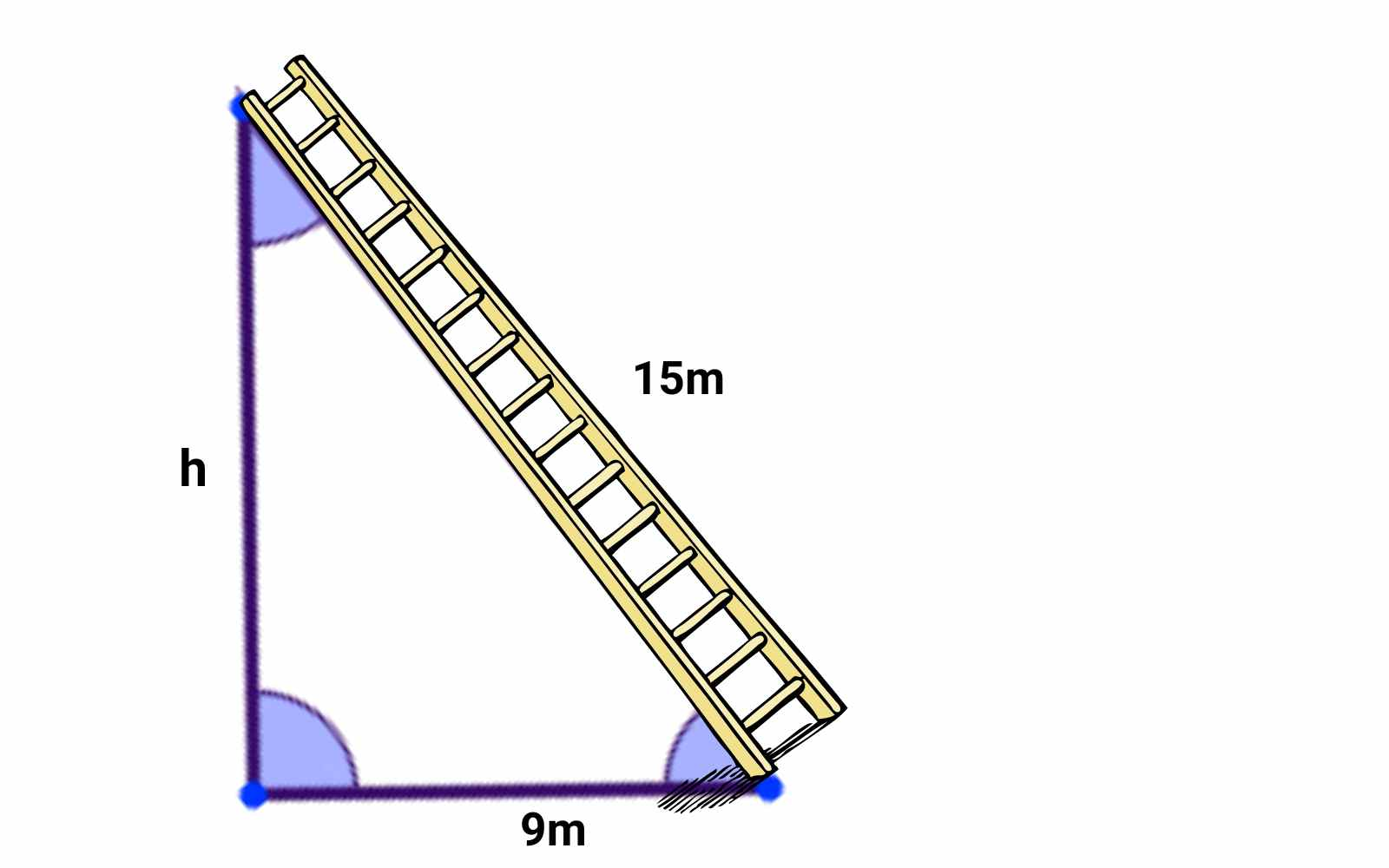Solution: We can use the Pythagorean Theorem to find the height at which the ladder touches the wall as follows:

Let the height at which the ladder touches the wall be “h.” Then, we can use the Pythagorean Theorem to find h as:

• a² + b² = c²
• 9² + h² = 15²
• 81 + h² = 225
• h² = 144
• h = √144 = 12 meters

Therefore, the ladder touches the wall at a height of 12 feet.

## Learn the Pythagoras Theorem Fast!

You know know that the Pythagoras Theorem is a maths theory that you can use to help solve questions about right triangles! It is one of the foundational concepts that will help you succeed in almost every maths subject. If you are currently struggling in maths, feel you need extra support or simply want to get an A in maths, A Team Tuition is here to help by providing one to one support.

Looking to improve your maths grade but unsure where to start? A Team Tuition is here to help. Our tried and true tutoring methods can help you improve in any subject with at-home and online tutoring. Find a maths tutor near you today!